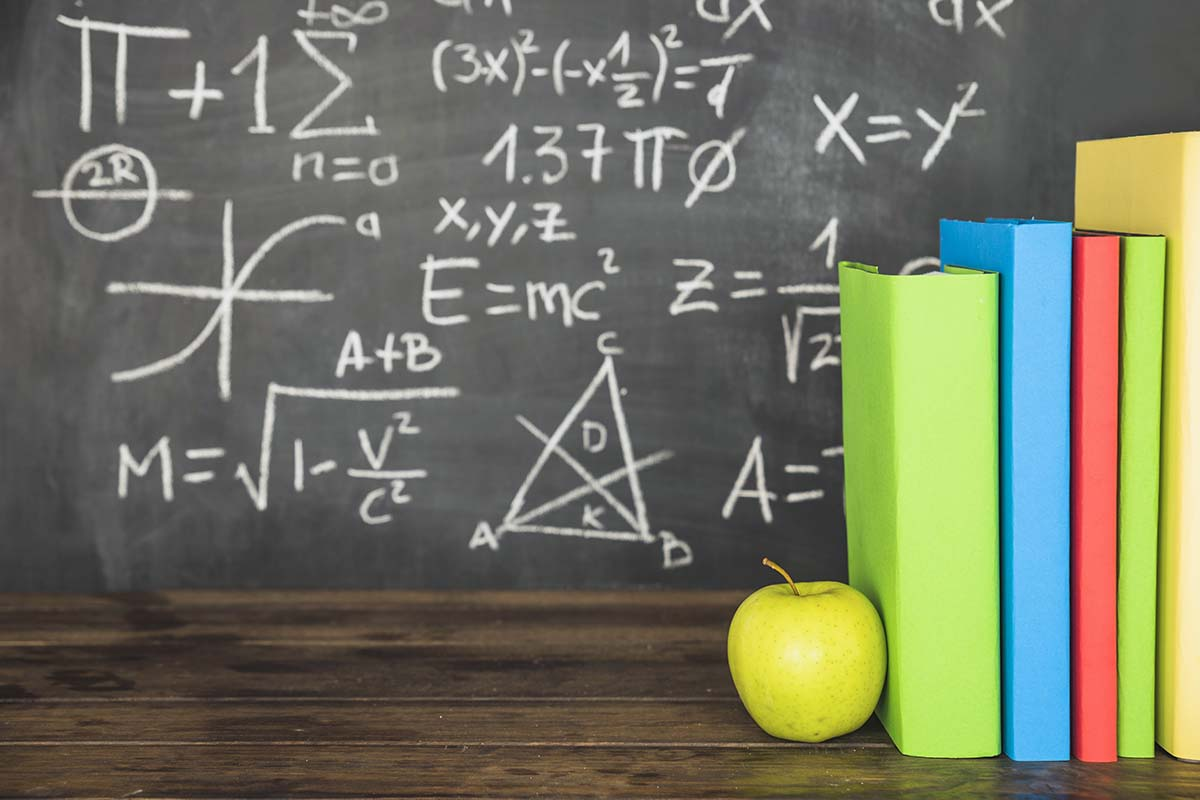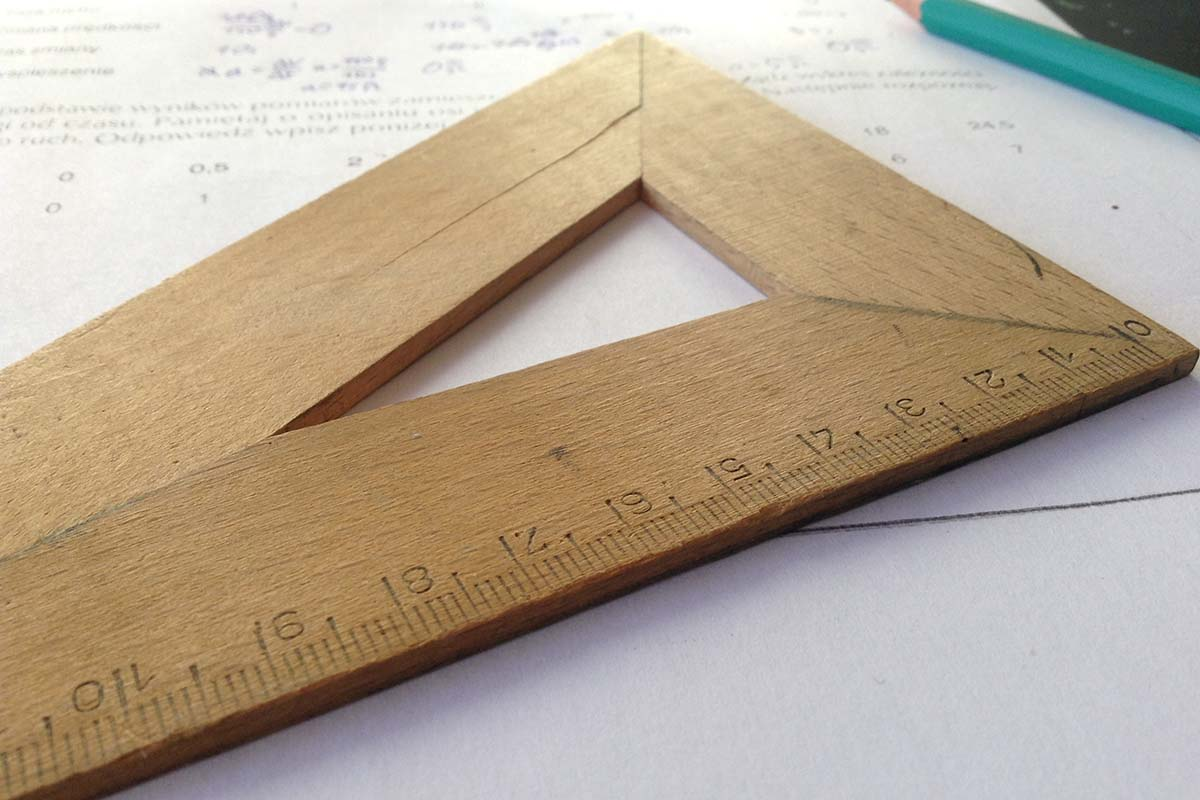# Here’s An Overview Of The Class 9 Maths Chapters

Written By Alla Levin
December 29, 2021## Here’s An Overview Of The Class 9 Maths Chapters

Class 9 is here, students have finished their Class 8 exams and are ready to move on to the next class. Students who are keen on getting full marks in Maths will also note that the concepts taught in their subjects get much more complex and harder, but such is the high school years. It is inevitable that students will have difficulty in getting accustomed to studying newer concepts in the next class, and this applies especially to students who will be studying new concepts in Mathematics.

So one of the best ways to get familiar with the new NCERT syllabus for Class 9 is by understanding what each and every chapter is going to offer a student in terms of difficulty.

This article will help a student by giving them a fair idea of what each and every chapter of Class 9 Maths has, which will further help them in understanding all the important concepts even before the class starts and give them some amount of fair knowledge about these chapters as compared to the other students who will be learning it fresh and new.

Contents

### Number Systems

The very first chapter of Class 9 Maths is Number Systems. This chapter will teach a student about the concept of rational and irrational numbers, real numbers and their decimal expansions, how to represent real numbers on the number line, and the laws of exponents for real numbers. This is one of the easier chapters in Class 9 NCERT Maths and students are expected to firmly grasp the meaning of its concepts in the first lecture itself.

### Polynomials

The second chapter is Polynomials. This chapter will guide students through different topics about polynomials like polynomials in one variable, the remainder theorem, the factorization of polynomials, and algebraic identities. The difficulty for this chapter is also rated to be lower than the others.

### Coordinate GeometryThis is where it starts to get interesting. Coordinate Geometry is going to be the first chapter in the NCERT Class 9 Maths textbook, which will require a student to work with visuals. Students will learn about the Cartesian System, and how to plot points in the Cartesian plane with coordinates.

### Linear Equations in Two Variables

The fourth chapter in the book will be a bit tricky. This chapter introduces students to the concept of Linear equations, how to solve a linear equation, and finding out the linear equation with the use of a graph. This chapter is also easy, but finding equations on a graph can get tricky.

### Introduction to Euclid’s Geometry

Here’s an interesting chapter about geometry. Students will be guided through topics about Euclid’s prowess in geometry, his definitions, axioms & postulates, the fifth postulate, and how the failed attempts to prove Euclid’s fifth postulate led to the discovery of other geometries known as non-Euclidian geometries.

### Lines and Angles

Students will learn about collinear and non-collinear points, intersecting line and non-intersecting lines,  pair of angles, Transversal point, lines parallel to the same line, and the angle sum property of a triangle.

### Triangles

Triangle is an easy chapter in terms of geometry in this chapter. Students will be taught about the congruence of triangles, the criteria (and some more) required for a triangle to be congruent, some properties of the triangle, and the inequalities in a triangle.

Just like triangles, students will learn about the angle sum property of a quadrilateral shape, properties of a parallelogram, and the mid-point theorem.

### Areas of Parallelograms and Triangles

This chapter will teach students about how to calculate areas of parallelograms and triangles, as the name says.

### Circles

Circles will also guide students through topics like angles subtended by a chord at a point, the perpendicular from the center to a chord, equal chords and their distance from the center, angles subtended by the arc of a circle, and cyclic quadrilaterals.

### Constructions

Topics for this chapter include how to construct a circle, perpendicular bisectors, triangles, etc. This chapter will require students to have a proper geometry set.

### Heron’s Formula

This chapter will teach students about finding out the area of a triangle when all three sides of a triangle are properly known.

### Surface Areas and Volumes

This is a hard chapter. Students will learn about finding the area of a cuboid and a cube and its formula.

### Statistics

This and the next chapter are the easiest of the 15 given chapters. This chapter teaches students how to collect, represent and also give a graphical representation of data.

### ProbabilityThis chapter is very simple, it teaches students how to calculate the probability of an event either happening or the chances of it not happening. Students should feel free to take help from various problem-solving books when studying Mathematics in Class 9. This will not only help them revise what they have learned, but students will also be able to become proficient in familiarizing themselves with the tougher questions that these books provide.

These questions are more complex and difficult because they ask questions that are not provided in the NCERT Maths book, these questions are made by the authors who kept in mind to make sure that the questions they publish should test the student’s ability to solve problems, this it does so by testing the knowledge of a student’s fundamental understanding of basic as well as difficult topics from the NCERT book. These books in turn help sharpen the mind of the student and give them a competitive edge compared to their peers.

An example of an amazing problem-solving book in the Class 9 RS Aggarwal Solutions (includes solutions for all chapters, like Chapter 1 & 2). RS Aggarwal’s problem-solving books for Mathematics are some of India’s most student-trusted books ever. These books provide a plethora of practice questions that students can devote their time to so that they learn how to solve even the toughest questions that appear in the exams. Another special benefit of practicing Maths from these books is that it has solutions to its answers too!